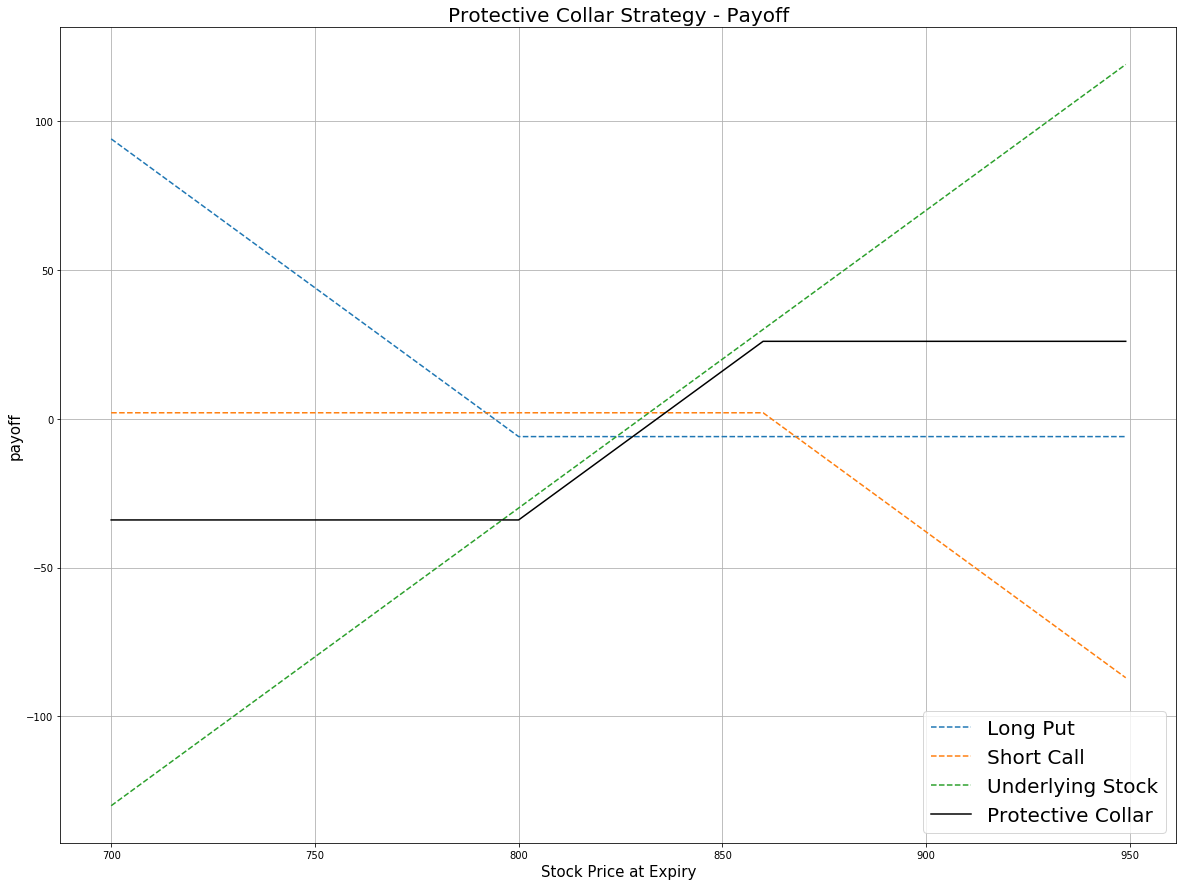# Option Strategies

## Protective Collar

### Introduction

A Protective Collar is an Options strategy that consists of a covered call and a long put (protective put) with a lower strike price than the short call contract. In contrast to the covered call, the protective put component limits the drawdown of the strategy when the underlying price decreases too much.

### Implementation

Follow these steps to implement the protective collar strategy:

1. In the Initialize method, set the start date, set the end date, subscribe to the underlying Equity, and create an Option universe.
2. private Symbol _equitySymbol, _optionSymbol;

public override void Initialize()
{
SetStartDate(2017, 4, 1);
SetEndDate(2017, 4, 30);
SetCash(100000);

_optionSymbol = option.Symbol;
option.SetFilter(universe => universe.Strikes(-10, 10)
.Expiration(TimeSpan.FromDays(0), TimeSpan.FromDays(30)));
}
def Initialize(self) -> None:
self.SetStartDate(2017, 4, 1)
self.SetEndDate(2017, 4, 30)
self.SetCash(100000)

self.option_symbol = option.Symbol
option.SetFilter(-10, +10, timedelta(0), timedelta(30))
3. In the OnData method, select the Option contracts.
4. public override void OnData(Slice slice)
{
if (Portfolio.Invested) return;

// Get the OptionChain
var chain = slice.OptionChains.get(_optionSymbol, null);
if (chain == null || chain.Count() == 0) return;

// Select an expiry date
var expiry = chain.OrderBy(x => x.Expiry).Last().Expiry;

// Select the call and put contracts that expire on the selected date
var calls = chain.Where(x => x.Expiry == expiry && x.Right == OptionRight.Call);
var puts = chain.Where(x => x.Expiry == expiry && x.Right == OptionRight.Put);
if (calls.Count() == 0 || puts.Count() == 0) return;

// Select the OTM contracts
var call = calls.OrderBy(x => x.Strike).Last();
var put = puts.OrderBy(x => x.Strike).First();
def OnData(self, slice: Slice) -> None:
if self.Portfolio.Invested: return

# Get the OptionChain
chain = slice.OptionChains.get(self.symbol, None)
if not chain: return

# Select an expiry date
expiry = sorted(chain, key = lambda x: x.Expiry)[-1].Expiry

# Select the call and put contracts that expire on the selected date
calls = [x for x in chain if x.Right == OptionRight.Call and x.Expiry == expiry]
puts = [x for x in chain if x.Right == OptionRight.Put and x.Expiry == expiry]
if not calls or not puts: return

# Select the OTM contracts
call = sorted(calls, key = lambda x: x.Strike)[-1]
put = sorted(puts, key = lambda x: x.Strike)
5. In the OnData method, submit the orders.
6. Sell(call.Symbol, 1);    // Sell the OTM call
Buy(_equitySymbol, 100); // Buy 100 shares of the underlying stock
self.Sell(call.Symbol, 1)          # Sell the OTM call
self.Buy(self.equity_symbol, 100)  # Buy 100 shares of the underlying stock

### Strategy Payoff

This is a limited-profit-limited-loss strategy. The payoff is

$$\begin{array}{rcll} C_T & = & (S_T - K^{C})^{+}\\ P_T & = & (K^{P} - S_T)^{+}\\ Payoff_T & = & (S_T - S_0 - C_T + P_T + C_0 - P_0)\times m - fee \end{array}$$ $$\begin{array}{rcll} \textrm{where} & C_T & = & \textrm{Call value at time T}\\ & P_T & = & \textrm{Put value at time T}\\ & S_T & = & \textrm{Underlying asset price at time T}\\ & K^{C} & = & \textrm{Call strike price}\\ & K^{P} & = & \textrm{Put strike price}\\ & Payoff_T & = & \textrm{Payout total at time T}\\ & S_0 & = & \textrm{Underlying asset price when the trade opened}\\ & C_0 & = & \textrm{Call price when the trade opened (credit received)}\\ & P_0 & = & \textrm{Put price when the trade opened (debit paid)}\\ & m & = & \textrm{Contract multiplier}\\ & T & = & \textrm{Time of expiration} \end{array}$$

The following chart shows the payoff at expiration:The maximum profit is $K^{C} - S_T + C_0 - P_0$. It occurs when the underlying price is at or above the strike price of the call at expiration.

The maximum profit is $S_T - K^{P} + C_0 - P_0$. It occurs when the underlying price is at or below the strike price of the put at expiration.

If the Option is American Option, there is risk of early assignment on the sold contract.

### Example

The following table shows the price details of the assets in the algorithm:

AssetPrice ($)Strike ($)
Call2.85845.00
Put6.00822.50
Underlying Equity at expiration843.19-

Therefore, the payoff is

$$\begin{array}{rcll} C_T & = & (S_T - K^{C})^{+}\\ & = & (843.19-845.00)^{+}\\ & = & 0\\ P_T & = & (K^{P} - S_T)^{+}\\ & = & (822.50-843.19)^{+}\\ & = & 0\\ Payoff_T & = & (S_T - S_0 - C_T + P_T + C_0 - P_0)\times m - fee\\ & = & (843.19-833.17+0-0+2.85-6.00)\times100-1.00\times3\\ & = & 684\\ \end{array}$$

So, the strategy gains \$684.

The following algorithm implements a protective collar Option strategy:

You can also see our Videos. You can also get in touch with us via Discord.